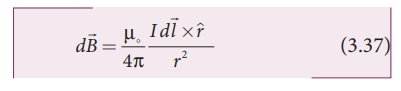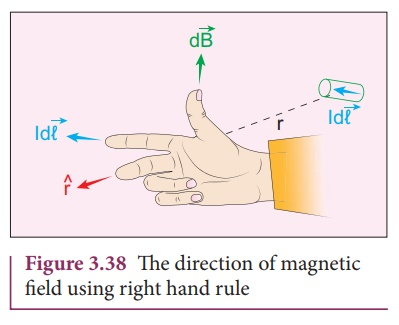Home | | Physics 12th Std | Definition and explanation of Biot- Savart law

# Definition and explanation of Biot- Savart law

Physics : Magnetism and Magnetic Effects of Electric Current

Definition and explanation of Biot- Savart law

Biot and Savart experimentally observed that the magnitude of magnetic field dat a point P (Figure 3.37) at a distance r from the small elemental length taken on a conductor carrying current variesi.directly as the strength of the current I

ii.directly as the magnitude of the length elementiii.directly as the sine of the angle (say,θ) betweenand r ^ .

iv.inversely as the square of the distance between the point P and length element.

This is expressed asin SI units and k = 1 in CGS units. In vector notation,Here vector dis perpendicular to both I(pointing the direction of current flow) and the unit vector r ^ directed fromtoward point P (Figure 3.38).The equation (3.37) is used to compute the magnetic field only due to a small elemental length dl of the conductor. The net magnetic field at P due to the conductor is obtained from principle of superposition by considering the contribution from all current elements I. Hence integrating equation (3.37), we getwhere the integral is taken over the entire current distribution.

Cases

1.     If the point P lies on the conductor, then θ = 0º. Therefore,is zero.

2.     If the point lies perpendicular to the conductor, then θ = 90º. Therefore,is maximum and is given bywhereis the unit vector perpendicular to both Iand.

Similarities between Coulomb’s law and Biot-Savort’s law

Electric and magnetic fields

·        obey inverse square law, so they are long range fields.

·        obey the principle of superposition and are linear with respect to source. In magnitude,Note that the exponent of charge q (source) and exponent of electric field E is unity. Similarly, the exponent of current element Idl (source) and exponent of magnetic field B is unity. In other words, electric fieldis proportional only to charge (source) and not on higher powers of charge ( q2 , q3 , etc). Similarly, magnetic fieldis proportional to current element I(source) and not on square or cube or higher powers of current element. The cause and effect have linear relationship.

Study Material, Lecturing Notes, Assignment, Reference, Wiki description explanation, brief detail
12th Physics : Magnetism and Magnetic Effects of Electric Current : Definition and explanation of Biot- Savart law |